## Monday, September 29, 2014

### Data Mining in Excel Part 22: More Forecasting

Today, we're going to talk some more about the Forecast algorithm within the Data Mining Add-in for Excel.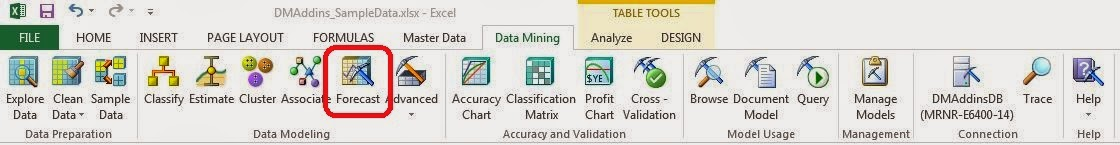Forecast
In the previous post, we built a simple forecasting algorithm to predict future sales values.  However, the model's predictions were not very good.  So, let's see if we can't use some of the parameters to create a better model.Parameters
Here are all the parameters we have access to.  For more information on what these parameters do, read this.  As we talked about in the previous post, this algorithm is made up of two distinct models, ARIMA and ARTXP.  The Prediction Smoothing parameter affects how much weight is applied to each model.  To get a starting point, let's set Prediction Smoothing to 0 and 1 to see how the pure ARIMA and pure ARTXP models fare.  To be clear, Prediction Smoothing = 0 is pure ARTXP and Prediction Smoothing = 1 is pure ARIMA.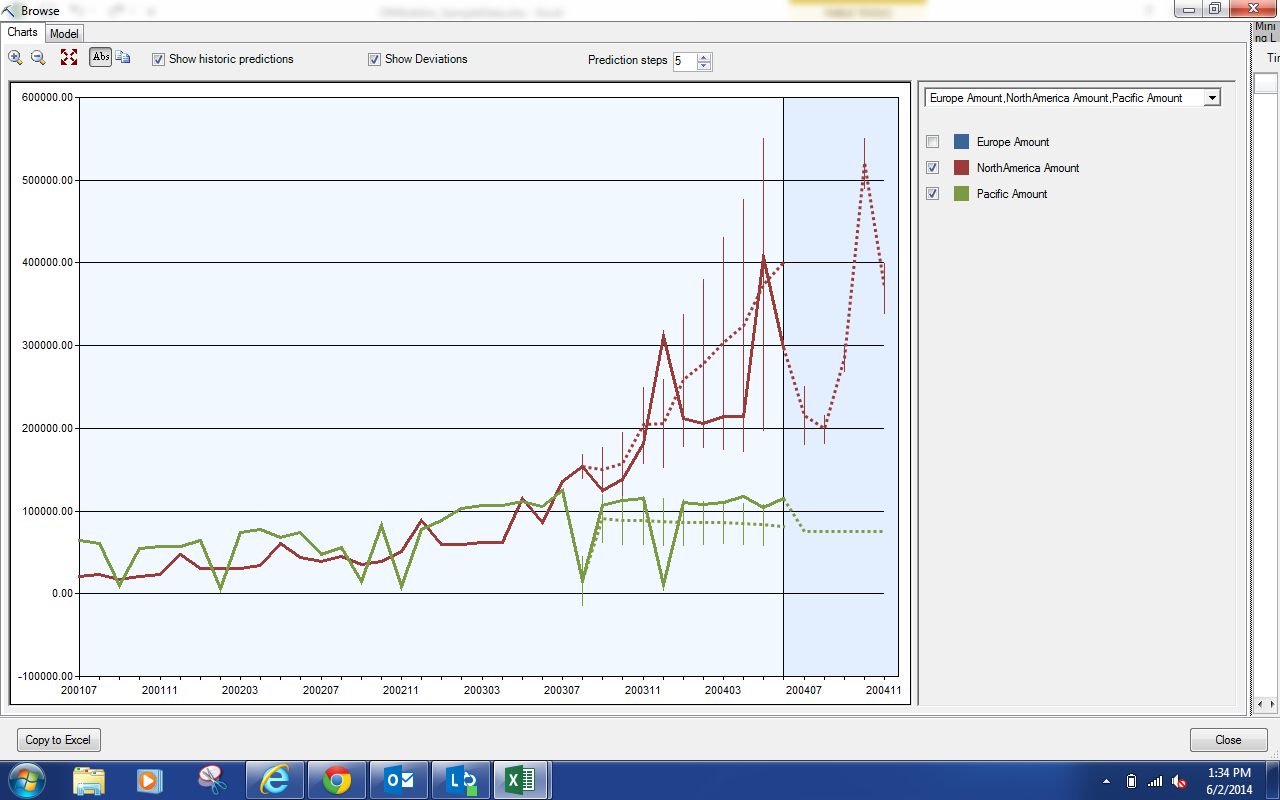ARTXP Model
The first thing we notice about this model is that the Confidence Bands (the vertical dotted lines) are enormous.  Far too big to have any confidence in a prediction.  This presents a very serious issue for using model.  Also, the model doesn't account for much of the variability across months.  Let's see how the ARIMA model fares.ARIMA Model
Immediately, we notice that the confidence bands on this model are extremely small, which is a good thing.  The model also seems to be accounting for the massive variability of the data.  However, the model is always choosing the wrong direction of gain or loss.  It thinks the value will go up when it actually goes down, and vice-versa.  We're pretty confident that we want to use the ARIMA model because of the tightness of the predictions; however, let's see if we can use some more parameters to finesse it a little.Parameters
Looking back at the parameters, we have Auto Detect Periodicity.  A periodicity is a distinct pattern over time in the data.  For instance, monthly data typically has a periodicity of 12 because customer habits tend to be similar around the same time every year.  On the same note, daily data might have periodicities of 7, 15 and 30 because that's when people get paid.  Many promotions begin and end on Tuesdays, which seems to create a weekly periodicity in retail/sales data.  Let's see what happens when we set this value to .3 and .8.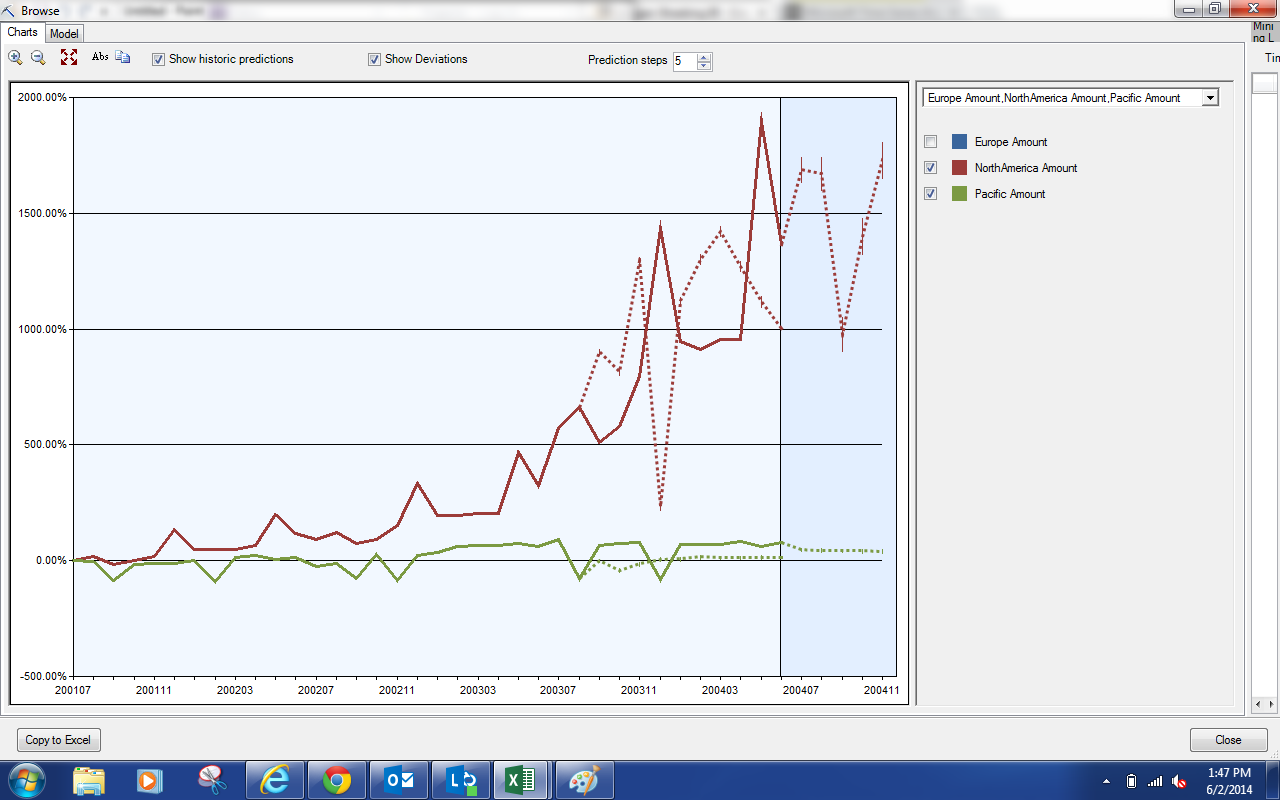ARIMA Model (.3 ADP)ARIMA Model (.8 ADP)
Looking at both of these models, we see no discernible difference.  For curiosity's sake, let's see what happens if we use Auto Detect Periodicity = 0.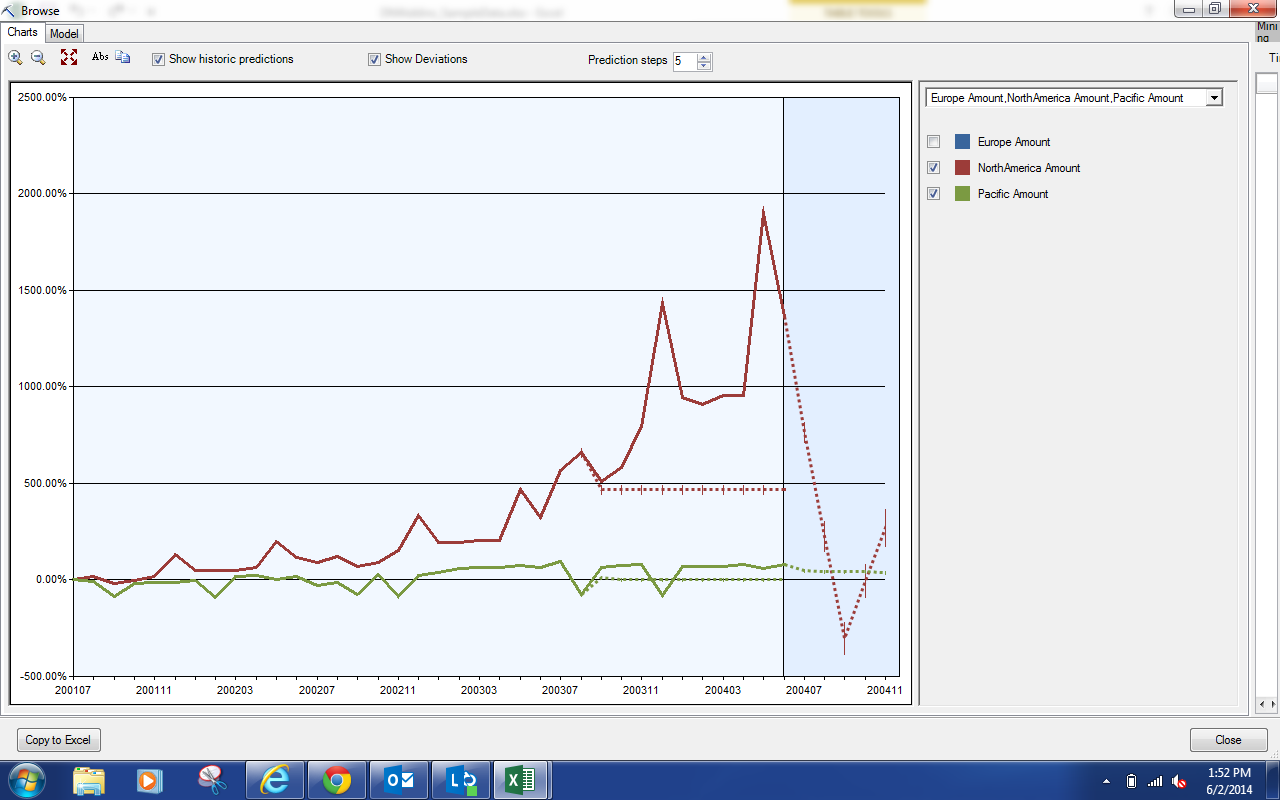ARIMA Model (0 ADP)
This model appears to be predicting using the overall mean or something like it.  It doesn't matter much because it doesn't seem that we can use this parameter to fix our issue.  Let's go back.Parameters
There's another periodicity parameter called Periodicity Hint.  This parameter allows you to tell the algorithm what kind of periodicity you think your data has.  Our data has some definite peaks that appear in the middle and at the end of every year.  So, why don't we set this value to {6, 12}.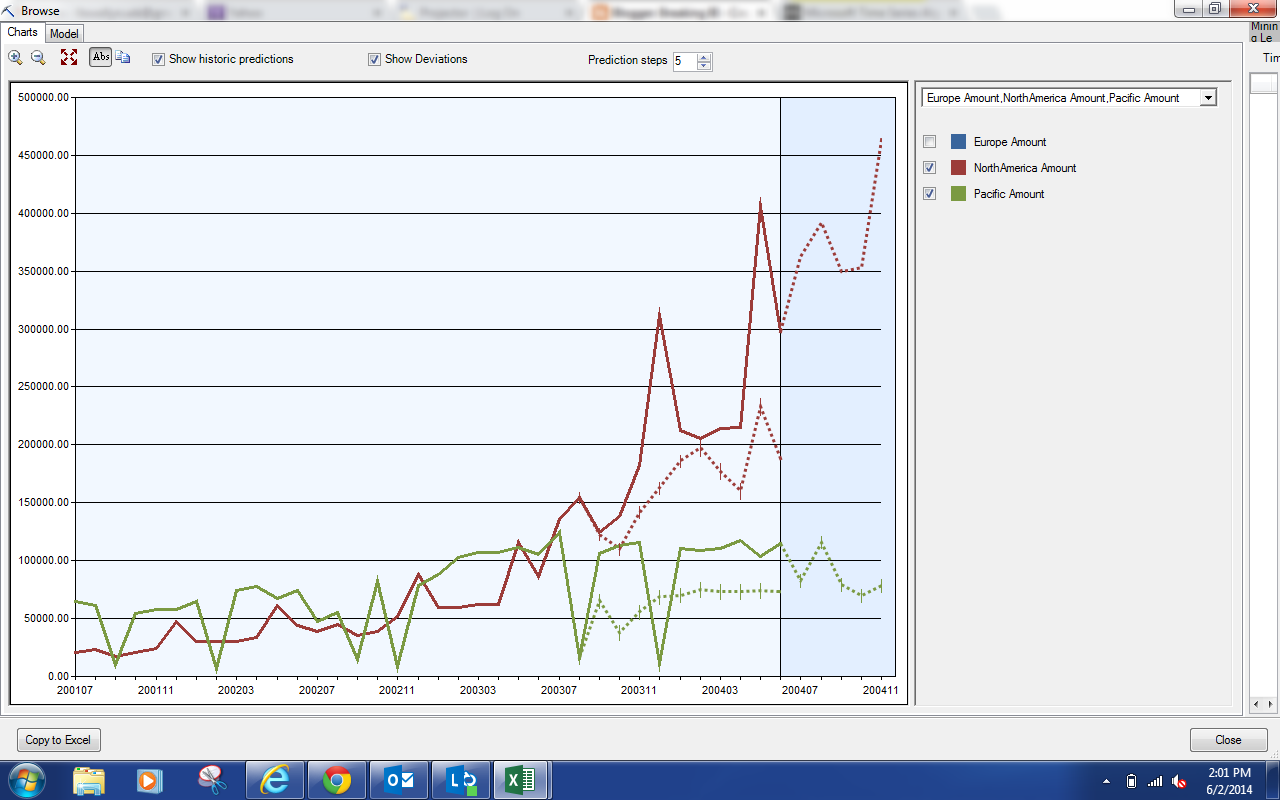ARIMA Model ({6, 12} PH)
This model seems to account for the highs and lows much better than the previous ones.  However, we have an issue that seems apparent now.  Our data has a very recent upward trend.  Our historic predictions don't seem to be accounting for this.  The historic prediction model is built using two parameters, Historic Model Count and Historic Model Gap.Parameters
Historic Model Count dictates how many distinct historic models are built and Historic Model Gap dictates how many months each model will predict.  For instance, the defaults were 1 and 10.  This means that 1 model was built for the previous 10 months, using only data from more than 10 months ago.  If we set these values to 2 and 5, we would get two models.  The first model would see data more than 10 months ago and predict data until 5 months ago.  The second model would see data more than 5 months ago and predict data until the final month.  This would let us see how the model predicts for observations in the near future.  Let's set these values to 3 and 2 and see what happens.ARIMA Model ({6, 12} PH, 3 HMC, 2 HMG)
As you can, these historic predictions are FAR better than what we had seen before.  This leads us to believe that our model is pretty good, but only for a short period into the future.  If we want to see further than that, we either need to use a different type of model (which would require a different tool) or get more data (which would require waiting).

This was a good investigation into the Microsoft Time Series Algorithm.  Stay tuned for our next post where we will be talking about creating multiple models under a single structure.  Thanks for reading.  We hope you found this informative.

Brad Llewellyn
Director, Consumer Sciences
Consumer Orbit
llewellyn.wb@gmail.com
http://www.linkedin.com/in/bradllewellyn

#### 1 comment:

1.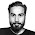Hi

I do know how the ARIMA model works and I have used it in SPSS and STATA. But how do we interpret this result from Excel? I mainly would like to know what was the ARIMA equations selected by excel and, if there is time, any other statistic of the process or the result.

ARIMA equation:

ARIMA ({1,-0.437850777450388,-0.275446162796555,-0.147157624359275,0.258456568312988},0,{1,0.40589820815716,6.92517291464772E-02,-0.562913240510376,-3.78748928313655E-02,0.764136397379578,0.361501798658526}) X ({1,0.89587094113018},1,{1})(6) Interceptar:35168.3950839853